Phase modulationCustom SearchPHASE MODULATION Frequency modulation requires the oscillator frequency to deviate both above and below the carrier frequency. During the process of frequency modulation, the peaks of each successive cycle in the modulated waveform occur at times other than they would if the carrier were unmodulated. This is actually an incidental phase shift that takes place along with the frequency shift in fm. Just the opposite action takes place in phase modulation. The af signal is applied to a PHASE MODULATOR in pm. The resultant wave from the phase modulator shifts in phase, as illustrated in figure 2-17. Notice that the time period of each successive cycle varies in the modulated wave according to the audio-wave variation. Since frequency is a function of time period per cycle, we can see that such a phase shift in the carrier will cause its frequency to change. The frequency change in fm is vital, but in pm it is merely incidental. The amount of frequency change has nothing to do with the resultant modulated wave shape in pm. At this point the comparison of fm to pm may seem a little hazy, but it will clear up as we progress. Figure 2-17. - Phase modulation.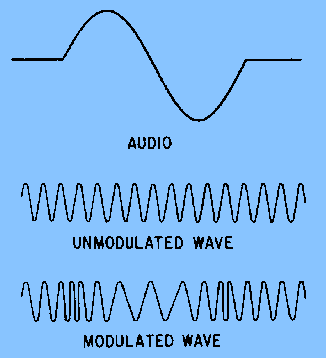Let's review some voltage phase relationships. Look at figure 2-18 and compare the three voltages (A, B, and C). Since voltage A begins its cycle and reaches its peak before voltage B, it is said to lead voltage B. Voltage C, on the other hand, lags voltage B by 30 degrees. In phase modulation the phase of the carrier is caused to shift at the rate of the af modulating signal. In figure 2-19, note that the unmodulated carrier has constant phase, amplitude, and frequency. The dotted wave shape represents the modulated carrier. Notice that the phase on the second peak leads the phase of the unmodulated carrier. On the third peak the shift is even greater; however, on-the fourth peak, the peaks begin to realign phase with each other. These relationships represent the effect of 1/2 cycle of an af modulating signal. On the negative alternation of the af intelligence, the phase of the carrier would lag and the peaks would occur at times later than they would in the unmodulated carrier. Figure 2-18. - Phase relationships.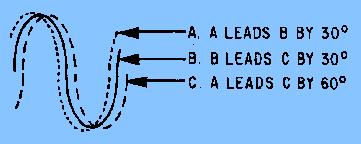Figure 2-19. - Carrier with and without modulation.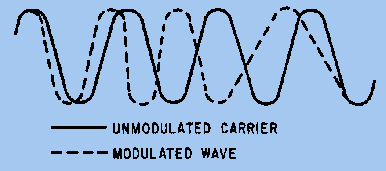The presentation of these two waves together does not mean that we transmit a modulated wave together with an unmodulated carrier. The two waveforms were drawn together only to show how a modulated wave looks when compared to an unmodulated wave. Now that you have seen the phase and frequency shifts in both fm and pm, let's find out exactly how they differ. First, only the phase shift is important in pm. It is proportional to the af modulating signal. To visualize this relationship, refer to the wave shapes shown in figure 2-20. Study the composition of the fm and pm waves carefully as they are modulated with the modulating wave shape. Notice that in fm, the carrier frequency deviates when the modulating wave changes polarity. With each alternation of the modulating wave, the carrier advances or retards in frequency and remains at the new frequency for the duration of that cycle. In pm you can see that between one alternation and the next, the carrier phase must change, and the frequency shift that occurs does so only during the transition time; the frequency then returns to its normal rate. Note in the pm wave that the frequency shift occurs only when the modulating wave is changing polarity. The frequency during the constant amplitude portion of each alternation is the REST FREQUENCY. Figure 2-20. - Pm versus fm.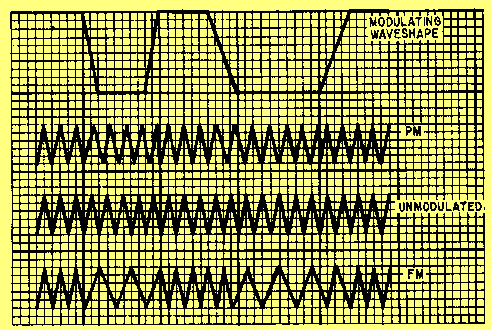The relationship, in pm, of the modulating af to the change in the phase shift is easy to see once you understand AM and fm principles. Again, we can establish two clear-cut rules of phase modulation: AMOUNT OF PHASE SHIFT IS PROPORTIONAL TO THE AMPLITUDE OF THE MODULATING SIGNAL. (If a 10-volt signal causes a phase shift of 20 degrees, then a 20-volt signal causes a phase shift of 40 degrees.) RATE OF PHASE SHIFT IS PROPORTIONAL TO THE FREQUENCY OF THE MODULATING SIGNAL. (If the carrier were modulated with a 1-kilohertz tone, the carrier would advance and retard in phase 1,000 times each second.) Phase modulation is also similar to frequency modulation in the number of sidebands that exist within the modulated wave and the spacing between sidebands. Phase modulation will also produce an infinite number of sideband frequencies. The spacing between these sidebands will be equal to the frequency of the modulating signal. However, one factor is very different in phase modulation; that is, the distribution of power in pm sidebands is not similar to that in fm sidebands, as will be explained in the next section. Modulation Index Recall from frequency modulation that modulation index is used to calculate the number of significant sidebands existing in the waveform. The higher the modulation index, the greater the number of sideband pairs. The modulation index is the ratio between the amount of oscillator deviation and the frequency of the modulating signal: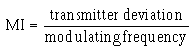In frequency modulation, we saw that as the frequency of the modulating signal increased (assuming the deviation remained constant) the number of significant sideband pairs decreased. This is shown in views (A) and (B) of figure 2-21. Notice that although the total number of significant sidebands decreases with a higher frequency-modulating signal, the sidebands spread out relative to each other; the total bandwidth increases. Figure 2-21. - Fm versus pm spectrum distribution.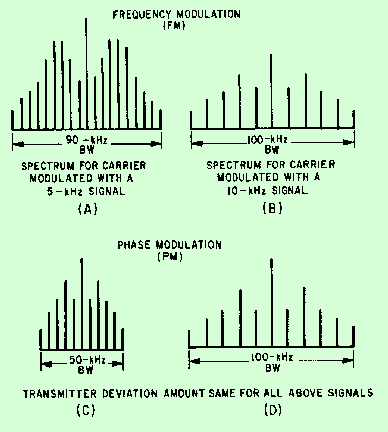In phase modulation the oscillator does not deviate, and the power in the sidebands is a function of the amplitude of the modulating signal. Therefore, two signals, one at 5 kilohertz and the other at 10 kilohertz, used to modulate a carrier would have the same sideband power distribution. However, the 10-kilohertz sidebands would be farther apart, as shown in views (C) and (D) of figure 2-21. When compared to fm, the bandwidth of the pm transmitted signal is greatly increased as the frequency of the modulating signal is increased.Integrated Publishing, Inc. - A (SDVOSB) Service Disabled Veteran Owned Small Business